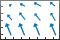# quiver

•## 语法

``quiver(X,Y,U,V)``
``quiver(U,V)``
``quiver(___,scale)``
``quiver(___,LineSpec)``
``quiver(___,LineSpec,'filled')``
``quiver(___,Name,Value)``
``quiver(ax,___)``
``q = quiver(___)``

## 说明

``quiver(X,Y,U,V)` 在由 `X` 和 `Y` 指定的笛卡尔坐标上绘制具有定向分量 `U` 和 `V` 的箭头。例如，第一个箭头源于点 `X(1)` 和 `Y(1)`，按 `U(1)` 水平延伸，按 `V(1)` 垂直延伸。默认情况下，`quiver` 函数缩放箭头长度，使其不重叠。`
````quiver(U,V)` 在等距点上绘制箭头，箭头的定向分量由 `U` 和 `V` 指定。 如果 `U` 和 `V` 是向量，则箭头的 x 坐标范围是从 1 到 `U` 和 `V` 中的元素数，并且 y 坐标均为 1。如果 `U` 和 `V` 是矩阵，则箭头的 x 坐标范围是从 1 到 `U` 和 `V` 中的列数，箭头的 y 坐标范围是从 1 到 `U` 和 `V` 中的行数。 ```

````quiver(___,scale)` 调整箭头的长度： 当 `scale` 为正数时，`quiver` 函数会自动调整箭头的长度，使其不重叠，然后将箭头长度拉伸 `scale` 倍。例如，`scale` 为 2 会使箭头长度加倍，`scale` 为 0.5 会使箭头长度减半。当 `scale` 为 `'off'` 或 `0` 时，如 `quiver(X,Y,U,V,'off')`，则禁用自动缩放。 ```

``quiver(___,LineSpec)` 设置线型、标记和颜色。标记出现在由 `X` 和 `Y` 指定的点上。如果使用 `LineSpec` 指定标记，则 `quiver` 不显示箭尖。要指定标记并显示箭尖，请改为设置 `Marker` 属性。`
``quiver(___,LineSpec,'filled')` 填充由 `LineSpec` 指定的标记。`
``quiver(___,Name,Value)` 使用一个或多个名称-值对组参数指定箭头图属性。有关属性列表，请参阅 Quiver 属性。在所有其他输入参数之后指定名称-值对组参数。名称-值对组参数应用于箭头图中的所有箭头。`

``quiver(ax,___)` 在 `ax` 指定的坐标区中而不是当前坐标区 (`gca`) 中创建箭头图。参数 `ax` 可以置于前面的语法中的任何输入参数组合之前。`

``q = quiver(___)` 返回 `Quiver` 对象。此对象对于在创建箭头图后控制其属性非常有用。`

## 示例

```load('wind','x','y','u','v') X = x(11:22,11:22,1); Y = y(11:22,11:22,1); U = u(11:22,11:22,1); V = v(11:22,11:22,1);```

```quiver(X,Y,U,V) axis equal``````[X,Y] = meshgrid(0:6,0:6); U = 0.25*X; V = 0.5*Y; quiver(X,Y,U,V,0)``````spacing = 0.2; [X,Y] = meshgrid(-2:spacing:2); Z = X.*exp(-X.^2 - Y.^2); [DX,DY] = gradient(Z,spacing);```

```quiver(X,Y,DX,DY) hold on contour(X,Y,Z) axis equal hold off``````[X,Y] = meshgrid(-pi:pi/8:pi,-pi:pi/8:pi); U = sin(Y); V = cos(X); quiver(X,Y,U,V,'r')``````[X,Y] = meshgrid(0:pi/8:pi,-pi:pi/8:pi); U1 = sin(X); V1 = cos(Y); U2 = sin(Y); V2 = cos(X); ```

```tiledlayout(1,2) ax1 = nexttile; quiver(ax1,X,Y,U1,V1) axis equal title(ax1,'Left Plot') ax2 = nexttile; quiver(ax2,X,Y,U2,V2) axis equal title(ax2,'Right Plot')``````[X,Y] = meshgrid(-pi:pi/8:pi,-pi:pi/8:pi); U = sin(Y); V = cos(X); q = quiver(X,Y,U,V); q.ShowArrowHead = 'off'; q.Marker = '.';```## 输入参数

`"-"`实线`"--"`虚线`":"`点线`"-."`点划线`"o"`圆圈`"+"`加号`"*"`星号`"."``"x"`叉号`"_"`水平线条`"|"`垂直线条`"square"`方形`"diamond"`菱形`"^"`上三角`"v"`下三角`">"`右三角`"<"`左三角`"pentagram"`五角形`"hexagram"`六角形`"red"``"r"``[1 0 0]``"green"``"g"``[0 1 0]``"blue"``"b"``[0 0 1]``"cyan"` `"c"``[0 1 1]``"magenta"``"m"``[1 0 1]``"yellow"``"y"``[1 1 0]``"black"``"k"``[0 0 0]``"white"``"w"``[1 1 1]`### 名称-值参数

• `'on'` - 显示带箭尖的向量。

• `'off'` - 显示不带箭尖的向量。

• `'on'` - 缩放箭头长度以便可容纳在网格定义的坐标数据和缩放箭头内，这样它们不会发生重叠。然后，`quiver``quiver3` 函数将 `AutoScaleFactor` 应用于箭头长度。

• `'off'` - 不缩放箭头长度。